# Electronics and Communication Engineering - Signals and Systems

Exercise : Signals and Systems - Section 1
46.
Which one is a causal system?
y(n) = 3x[n] - 2x[n - 1]
y(n) = 3x[n] + 2x[n + 1]
y(n) = 3x[n + 1] + 2x[n - 1]
y(n) = 3x[n + 1] 2x[n - 1] + x[n]
Explanation:

For causal output must depend upon Present and past not on future.

47.
If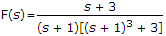, the coefficient of term e-t in f(t) will be
1
0
0.5
2/3
Explanation:

Coefficient of e-t =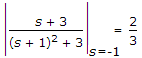.

48.
Double integration of a unit step function would lead to
an impulse
a parabola
a ramp
a doublet
Explanation: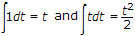.

49.
If f(t) = A d(t - a), F(s) is
A e-as
A eas
A a e-as
Aa eas
Explanation:

It is an impulse having area A and originating at t = a.

50.
If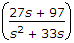is the Laplace transform of f(t) then f(0+) is
0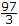27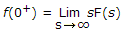.